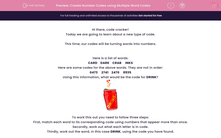# Create Number Codes using Multiple Word Codes

In this worksheet, students will turn words into number codes using multiple words and codes to find and apply the pattern. It will develop their encoding and problem solving skills.This content is premium and exclusive to EdPlace subscribers.Key stage:  KS 2

Curriculum topic:   Verbal Reasoning

Curriculum subtopic:   Number Code Breaking

Difficulty level:#### Worksheet Overview

Hi there, code cracker!

Today we are going to learn about a new type of code.

This time, our codes will be turning words into numbers.

Here is a list of words:

CARD    DARK    CRAB     INKS

Here are some codes for the above words. They are not in order:

0473     2741    2470     8935

Using this information, what would be the code for DRINK?To work this out you need to follow three steps:

First, match each word to its corresponding code using numbers that appear more than once.

Secondly, work out what each letter is in code.

Thirdly, work out the word, in this case DRINK, using the code you have found.

So let’s give it a try!

There are two codes beginning with the number 2. Do we have any words starting with the same letter?

We have two words starting with the letter C, so 2 must represent C (2=C).

We have two codes with the number 4 in the second letter position. Which letter appears in the second letter position twice? 4 must be the letter A (4=A).

We now have enough information to match each word to a code, giving us:

DARK = 0473

CRAB = 2741

CARD = 2470

INKS = 8935

So D = 0, R = 7, I = 8, N = 9, K = 3.

If we use this code on the word DRINK, we get the answer of 07893.

In this activity, you will be a code cracker and work out the digit-based code for a given word.

It can be really helpful to write the words out and then write the corresponding numbers above each letter as you work them out.  Give it a go!

Let’s get started!

### What is EdPlace?

We're your National Curriculum aligned online education content provider helping each child succeed in English, maths and science from year 1 to GCSE. With an EdPlace account you’ll be able to track and measure progress, helping each child achieve their best. We build confidence and attainment by personalising each child’s learning at a level that suits them.

Get started